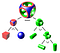# Crash course on CAD data. Part 2.

Previous parts

Part 1. How did this all begin?

# How is 3D data presented in CAD?

There can be multiple approaches to describing 3D data. Depending on problem domain and required operations on such data, the choice can be among the following options:

• Voxels;
• Meshes;
• CSG (Constructive Solid Geometry);
• B-Rep (Boundary Representation).

# Voxels

Voxel, or volume element, a 3D counterpart of a 2D pixel, can be found in medicine and scientific applications, and games to represent graphical objects on a scene via a set of fine-grained 3D elements. Voxel data model, for instance, can result from space scanning and attributing certain graphical property (such as color and transparency) to each small cell of a 3D grid.

# Meshes

Mesh is a tessellated (also known as facetted or polygonal) representation of a 3D model. Meshes can be:

• 1D: free-form curve approximation with linear segments;
• 2D: approximation of a surface with triangles, quadrangles or arbitrary polygons;
• 3D: filling-in the internal body space with tetrahedrons, hexahedrons, prisms, pyramids and others.

Most of the time, meshes are of the first order (i.e. mesh cells boundaries are either linear or planar). Some mesh generators are able to produce 2nd order elements. The former are more wide-spread due to reduced memory footprint and faster computations thereon. The latter allow more precise approximation at the expense of speed and footprint.

# CSG (Constructive Solid Geometry)

This technique describes a 3D body via a tree of (binary, Boolean) operations (such as fuse, common or cut) required to construct such a body.CSG objects can be represented by binary trees (image from Wikipedia)

# B-Rep (or Boundary Representation)

This technique describes a 3D body using its boundaries, namely:

• surfaces defining the body boundary in 3D space;
• curves defining intersections of above surfaces;
• points defining intersections of above curves.

B-Rep distinguishes geometry (which is used to describe the underlying curves and surfaces) and topology (which is used to describe boundaries that limit the geometry and connectivity information). We will talk more of those two concepts later in the series.

# Wireframe representation

It is a particular case of B-Rep representation used to describe 1D bodies in 3D, i.e. bodies defined via curves without surfaces. (When I was studying computational geometry and computer graphics in the University this was taught as an independent mechanism but in my professional life it turned out to be just a subtype of B-Rep, so I won’t make a distinction below).

In the CAD/CAM/CAE world, two of the above — B-Rep and meshes — have found the broadest use. Of course, each CAD system tries to add its own data in order to provide maximum data richness and context (such as history of body construction operations, their parameters, constraints and so on). But B-Rep and mesh remain two common denominators used to express 3D data in CAD/CAM/CAE and exchange between the systems.

We are going to talk more of these two in the following installment of the series. Stay tuned ;)

Next parts:

Part 3. Precise geometry and tessellation. B-Reps and meshes

Part 4. Parts and assemblies